# Quiz 12: Activity-Based Management

1.Value added costs: Value added costs are those generated because of value added activities which are necessary to remain in the business. Value added activities contribute to customer value and/or help meet and organization's needs. Value added costs can be calculated with the formulae,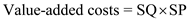Where, SQ = Value-added output level for an activity. SP = Standard price per unit of activity output measure Non-Value added costs: Non-Value added costs are those generated because of non-value added activities which are unnecessary and are not valued by internal or external customers. Non-value added activities often are those that fail to produce a change in state or those that replicate work because it wasn't done correctly the first time. Non-value added costs can be calculated using the formulae,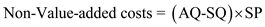Where, AQ = Actual quantity used of flexible resources or the practical activity acquired for committed resources. Total Cost: Total cost includes both value and non-value added costs. Cost report for showing Value and Non-value added activities and total cost: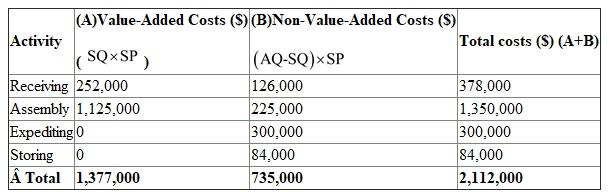The Value-added costs (A), Non-value added costs (B) and Total costs in the above table are obtained by the following way,Here, the values of SQ, AQ and SP are given in the question. The total is obtained by adding all the costs involved in the four activities. 2.Expediting products and Storing goods are non-value added activities because these are unnecessary and are not valued by internal or external customers. Non-value added activities often are those that fail to produce a change in state or those that replicate work because it wasn't done correctly the first time. 3.For Receiving, cost reduction occurs only when the actual demand for receiving orders is reduced by each block of 1,500 orders. For Assembly, each order reducing cost of waste produces a saving of \$15. Accordingly, reducing costs of wastes will likely materialize more quickly for Assembly than for receiving.
1.Non-value added costs can be calculated using the formulae,Cost report for showing Non-value added activities cost for 2013: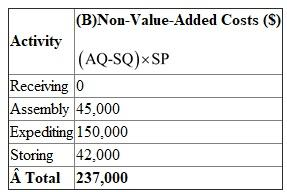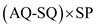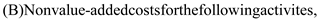The values of SQ, AQ and SP are given in the question. The total is obtained by adding the costs of the four activities Trend report of Non-Value-Added Costs: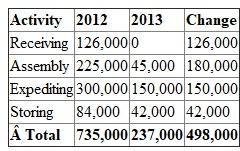The Non-value added costs in the column 2012 are the results obtained in the question Cornerstone Exercise 12.1.The difference of Non-value added costs reports in 2012 and 2013 is the result of the change in costs. The trend report shows a significant reduction in non-value-added costs, validating the improvement actions taken. 2.For value-added activities, the non-value-added component is usually the result of using more of the activity than should be used; thus, activity reduction is the objective for improving activity efficiency. For non-value-added activities, activity reduction is an intermediate step that will ultimately lead to activity elimination. Depending on the nature of resources consumed by the activity, activity reduction can also lead to cost reductions. 3.From requirement 1, the savings per unit of product are \$24.9 (\$498,000/20,000), indicating that the competitor's price reduction cannot be matched because there is a change with a difference of \$2.10 (\$27-\$24.9). Therefore, there is a need of change in the unit profit margin existed beginning of the year.